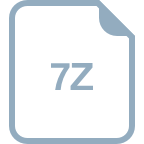# 帮我写一段实现电子时钟的C51代码

### 回答1： 好的，以下是实现电子时钟的C51代码： ``` #include <reg51.h> #include <intrins.h> #define uint unsigned int #define uchar unsigned char sbit SCL = P1^0; // I2C时钟线 sbit SDA = P1^1; // I2C数据线 uchar code table = {0x3f, 0x06, 0x5b, 0x4f, 0x66, 0x6d, 0x7d, 0x07, 0x7f, 0x6f}; // 数码管的码表 void I2CStart() { SDA = 1; SCL = 1; _nop_(); SDA = 0; _nop_(); SCL = 0; } void I2CStop() { SDA = 0; SCL = 1; _nop_(); SDA = 1; } void I2CSendByte(uchar dat) { uchar i; for(i=0; i<8; i++) { SDA = (dat & 0x80) >> 7; dat <<= 1; SCL = 1; _nop_(); SCL = 0; } SDA = 1; SCL = 1; _nop_(); SCL = 0; } void Display(uchar add, uchar dat) { I2CStart(); I2CSendByte(add); I2CSendByte(dat); I2CStop(); } void main() { uchar i; uint time; while(1) { for(i=0; i<=9; i++) { Display(0x40, table[i]); for(time=0; time<60000; time++); } } } ``` 注意：这段代码仅实现了数码管的循环显示，并不是完整的电子时钟代码，您可以根据需要进行修改。 ### 回答2： C51是一种常用的单片机，用于嵌入式系统的开发。下面是一个简单实现电子时钟的C51代码示例： ```c #include <reg52.h> // 定义全局变量 sbit RS = P2^2; // 控制LCD的寄存器选择 sbit RW = P2^1; // 控制LCD的读写 sbit E = P2^0; // 使能LCD sfr LCD_DATA = 0x90; // LCD数据端口 // 定义函数 void delay(unsigned int time) { while(time--); } void lcdWriteCmd(unsigned char cmd) { RW = 0; // 设置为写模式 RS = 0; // 选择控制命令 LCD_DATA = cmd; // 发送命令 E = 1; // 上升沿产生脉冲 delay(5); E = 0; } void lcdWriteData(unsigned char dat) { RW = 0; // 设置为写模式 RS = 1; // 选择数据 LCD_DATA = dat; // 发送数据 E = 1; // 上升沿产生脉冲 delay(5); E = 0; } void lcdInit() { lcdWriteCmd(0x38); // 8位数据接口，2行显示，5x7点阵 lcdWriteCmd(0x0C); // 显示器开，光标关闭 lcdWriteCmd(0x06); // 指针右移，显示不移动 lcdWriteCmd(0x01); // 清屏指令 } void lcdShowTime(unsigned char hour, unsigned char min, unsigned char sec) { lcdWriteCmd(0x80); // 指定显示位置为第一行第一列 lcdWriteData(hour/10 + 0x30); // 显示十位数 lcdWriteData(hour%10 + 0x30); // 显示个位数 lcdWriteData(':'); // 显示冒号 lcdWriteData(min/10 + 0x30); // 显示十位数 lcdWriteData(min%10 + 0x30); // 显示个位数 lcdWriteData(':'); // 显示冒号 lcdWriteData(sec/10 + 0x30); // 显示十位数 lcdWriteData(sec%10 + 0x30); // 显示个位数 } void main() { unsigned char hour, min, sec; lcdInit(); // 初始化LCD hour = 12; // 初始小时数为12 min = 0; // 初始分钟数为0 sec = 0; // 初始秒数为0 while(1) { lcdShowTime(hour, min, sec); // 在LCD上显示时间 sec++; // 秒数自增 if(sec == 60) { // 秒数达到60时 sec = 0; min++; // 分钟数自增 } if(min == 60) { // 分钟数达到60时 min = 0; hour++; // 小时数自增 } if(hour == 24) { // 小时数达到24时，回到0 hour = 0; } delay(50000); // 延时一秒钟 } } ``` 这段代码使用C51单片机实现了一个简单的电子时钟。它通过控制LCD模块显示current time。代码中的main函数通过不断自增时、分、秒数，并将其显示在LCD上，模拟了一个时钟的运作。同时，代码还实现了LCD的初始化以及控制LCD的命令和数据写入。通过调用函数，可以将时间显示在第一行，格式为"hh:mm:ss"。 请注意，上述代码仅为示例，并未经过实际测试，可能存在错误或不足之处。在实际应用中，还需根据具体硬件环境和LCD模块的规格参数进行适配和修改。 ### 回答3： C51是一种经典的单片机，用于嵌入式系统开发。下面是一个基本的C51代码来实现一个简单的电子时钟。 #include <reg52.h> // 引入单片机特定的寄存器定义 sbit led=P1^0; // 使用P1.0引脚作为LED显示 void main() { unsigned char hour=12, minute=0, second=0; // 初始化小时、分钟和秒钟变量 TMOD = 0x01; // 设置定时器0为工作模式1 TH0 = 0x3C; // 设置定时器0的高8位初值，每隔50ms中断一次 TL0 = 0xAF; // 设置定时器0的低8位初值 ET0 = 1; // 允许定时器0中断 EA = 1; // 允许总中断 TR0 = 1; // 启动定时器0 while(1) { led = ~led; // LED显示取反（闪烁） // 显示小时、分钟和秒钟在LED上 P0 = hour/10; // 十位 delay(); P0 = hour%10; // 个位 delay(); P0 = minute/10; // 十位 delay(); P0 = minute%10; // 个位 delay(); P0 = second/10; // 十位 delay(); P0 = second%10; // 个位 delay(); second++; // 秒钟加1 if (second >= 60) { second = 0; minute++; // 分钟加1 if (minute >= 60) { minute = 0; hour++; // 小时加1 if (hour >= 24) { hour = 0; } } } } } void delay() { unsigned int i,j; for (i=0;i<100;i++) { for (j=0;j<100;j++){} } } 上述代码中，我们使用了定时器0中断来控制电子时钟的时间显示和闪烁。我们定义了全局变量hour、minute和second来保存当前时间，并在while循环中进行时间的更新和LED的显示。同时，我们也定义了一个延迟函数delay()用于控制LED的闪烁频率。 通过该代码，我们可以在C51中实现一个基本的电子时钟，能够在LED上显示当前的小时、分钟和秒钟，并进行闪烁。注意，这只是一个简单的示例代码，实际的电子时钟可能还需要更复杂的功能和显示方式。

## 相关推荐